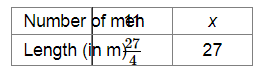# Solve the following`
Question:

11 men can dig $6 \frac{3}{4}$ metre long trench in one day. How many men should be employed for digging 27 metre long trench of the same type i one day?

Solution:

Let x be the number of men required to dig a trench of 27 metre.Since the length of the trench and the number of men are in direct variation, we have:

$\frac{11}{x}=\frac{27 / 4}{27}$

$\Rightarrow 11 \times 27=x \times \frac{27}{4}$

$\Rightarrow x=\frac{11 \times 27 \times 4}{27}$

$=44$

Thus, 44 men will be required to dig a trench of 27 metre.